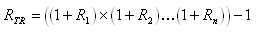Time-Weighted Return

The yield that the investment item itself has achieved.  This is the intrinsic performance, ignoring the timing of any external cash flows.  Use this return when creating or comparing against advertised performance numbers.  See Yield Calculations for a discussion of the differences between ROI and TWR yields.  TWR can be displayed for any investment, symbol, asset type, investment goal, sector, investment type, currency, or sub-portfolio.  The formula for calculating the true time-weighted return is:where EMVi is the market value at the end of sub-period i, excluding any cash flows in the period.  BMVi is the market value at the end of the previous sub-period (i.e., the beginning of the current sub-period), plus any cash flows at the end of the previous sub-period, where an inflow is positive and an outflow is negative.  The cash inflow is included in the BMV (previous period EMV + positive cash inflow) of the sub-period when the cash inflow is available for investment at the start of the sub-period; a cash outflow is reflected in the BMV (previous period EMV + negative cash outflow) of the sub-period when the cash outflow is no longer available for investment at the start of the sub-period.

The sub-period returns are then geometrically linked to calculate the period’s total return according to the following formula:where RTR is the period’s total return and R1, R2 Rn are the sub-period returns for sub-period 1 through n respectively.

Annualizing:

By default, yields for terms less than one year are not annualized, and yields for terms greater than one year are average annualized.  Whether or not to average annualize yields can be controlled from the Preferences - Yield dialog.

Accrued Interest:

By default, accrued interest is included in yield calculations.  Accrued interest for the beginning and ending of each sub-period is added into BMV / EMV.  Whether or not to include accrued interest in the yield calculations can be controlled from the Preferences - Yield dialog.

Example Calculation:
Assume we are calculating the 6 month (non-annualized) TWR ending on 12/31/2009.  The date range for this yield term would be 7/1/2009 through 12/31/2009, inclusive of gains on both the starting and ending dates.  The following hypothetical details are used in this example:

Market value at beginning of 7/1/09: \$1,000

Market value at end of 8/13/09 was \$1,200 before the new purchase, market value was \$2,400 after purchase

Received a cash dividend on 9/30/09 for \$50

Market value at end of 9/30/09 was \$2,500 (not counting the dividend)

Market value at end of 12/31/09: \$2,600

No accrued interest.

BMV1 = 1000; EMV1 = 1200; R1 = 20%

BMV2 = 2400; EMV2 = 2550; R2 = 6.25%

BMV3 = 2500; EMV3 = 2600; R3 = 4%

and we then combine the sub-period returns together, to get the total period return:

RTR = (1.2 x 1.0625 x 1.04) - 1 = 32.6%

Logging Details:

Fund Manager can optionally log the TWR calculation details to an external log file.  See General Preferences / Yields.